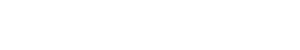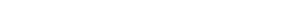## Quicksort Algorithm Implementation with Python

QuickSort is an O(nlogn) efficient sorting algorithm, serving as systematic method for placing elements of an array in order. Quicksort is a comparison sort, meaning that it can sort items of any type for which a “less-than” relation (formally, a total order) is defined. In efficient implementations it is not a stable sort, meaning that the relative order of equal sort items is not preserved. Quicksort can operate in-place on an array, requiring small additional amounts of memory to perform the sorting. It is very similar to selection sort, except that it does not always choose worst-case partition.
Source: https://en.wikipedia.org/wiki/Quicksort
Below are 2 versions of the Quicksort Algorithm Implementation with Python. The first version is easy, but use more memory. The second version use the memory very efficiently.

I- QuickSort Algorithm Implementation with Python (Memory intensive version)

Output:II- Quicksort Algorithm Implementation with Python (with Memory Optimisation)III- QuickSort Algorithm Implementation with Python for Both Methods with duration capturedOutputIII- Most Efficient Quicksort implementation in Python on array of  integer represented as string. Example: Array=[“1″,”237373737″,”3″,”1971771717171717″,”0”]

def QuickSort(array):
return sorted(array, key=lambda x: (len(x),x))
input:
```6
31415926535897932384626433832795
1
3
10
3
5```
Output:
```1
3
3
5
10
31415926535897932384626433832795

Just to clarify that `lambda` part, in case someone else doesn't understand how exactly string comparison works: `'2' > '1'` is `True`, but `'2' > '10'` is also `True`, as well as `'2' > '1000'`. That's why the strings are sorted by length first, because `len('2') < len('10')`.

IV- Build up a sorted array, one element at a time. Print the array after each iteration of the insertion sort, i.e., whenever the next element has been inserted at its correct position

```